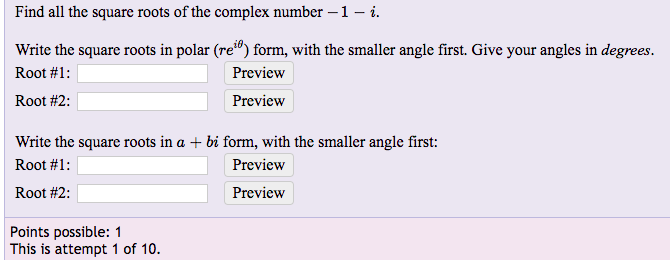Find all the square roots of the complex number-1-iWrite the square roots in polar (re0) form, with the smaller angle first. Give your angles in degreesRoot #1 :Root #2:PreviewPreviewWrite the square roots in a + bi form, with the smaller angle first:Root #1 :Root #2:PreviewPreviewPoints possible: 1This is attempt 1 of 10

Question

Find all the square roots of the complex number −1−i

Write the square roots in polar (re) form, with the smaller angle first. Give your angles in degrees.
Root #1:
Root #2:

Write the square roots in a+bi form, with the smaller angle first:
Root #1:
Root #2:

* PHOTO INCLUDED BELOWhelp_outlineImage TranscriptioncloseFind all the square roots of the complex number-1-i Write the square roots in polar (re0) form, with the smaller angle first. Give your angles in degrees Root #1 : Root #2: Preview Preview Write the square roots in a + bi form, with the smaller angle first: Root #1 : Root #2: Preview Preview Points possible: 1 This is attempt 1 of 10 fullscreen
Step 1

The complex number is z = – 1 – i, its square root give another complex number. Hence, it can be written as

Step 2

Find the exponential form of the complex number – 1 – i as follows.

Step 3

Thus, the exponential...

Want to see the full answer?

See Solution

Want to see this answer and more?

Our solutions are written by experts, many with advanced degrees, and available 24/7

See Solution
Tagged in

Trigonometric Ratios